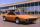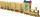Journey 5

A man has to do a journey of 84km in 3 hours. He travels the first 30km at 20km/hr. At what rate must he travel the remaining distance to complete his journey on time?

Result

v =  36 km/h

Solution:

84 - 30 = v * (3 - 30/20)

30v = 1080

v = 36

Calculated by our simple equation calculator.

Leave us a comment of this math problem and its solution (i.e. if it is still somewhat unclear...):Be the first to comment!To solve this verbal math problem are needed these knowledge from mathematics:

Do you have a linear equation or system of equations and looking for its solution? Or do you have quadratic equation?

Next similar math problems:

1. Theorem proveWe want to prove the sentence: If the natural number n is divisible by six, then n is divisible by three. From what assumption we started?
2. Flowers 2Cha cruz has a garden. The ratio roses to tulips is 2 : 5, the ratio of roses to orchids is 7 : 6. Cha cruz wonders what the ratio of tulips to orchids is. If Cha cruz has 183 plants, how many of each kind are there?
3. SpeedometerThe speedometer of a car reads 66 km/h. It's accurate to within 4.1% What is the maximum possible error?55%+36%+88%+71%+100=63% what is whole (X)? Percents can be added directly together if they are taken from the same whole, which means they have the same base amount. .. . You would add the two percentages to find the total amount.
5. Warehouse carsFrom the warehouse started truck at speed 40km/h. After 1hour 30mins started from the same place same direction a car at speed 70 km/h. For how long and at what distance from the warehouse overtake a truck?
6. AsymptoteWhat is the vertical asymptote of ?
7. Today in schoolThere are 9 girls and 11 boys in the class today. What is the probability that Suzan will go to the board today?
8. ChildrenThe group has 42 children. There are 4 more boys than girls. How many boys and girls are in the group?
9. EquationHow many real roots has equation ? ?
10. SchoolSchool attend 792 children, boys and girls ratio is 4:5. How many more girls go to school (than boys)?
11. ExaminationThe class is 21 students. How many ways can choose two to examination?
12. Three unknownsSolve the system of linear equations with three unknowns: A + B + C = 14 B - A - C = 4 2A - B + C = 0
13. Solve 3Solve quadratic equation: (6n+1) (4n-1) = 3n2
14. Percentage 1052 shorts and missed 13. Calculate percentage
15. TrigonometryIs true equality? ?
16. SequenceFind the common ratio of the sequence -3, -1.5, -0.75, -0.375, -0.1875. Ratio write as decimal number rounded to tenth.
17. LegsCancer has 5 pairs of legs. The insect has 6 legs. 60 animals have a total of 500 legs. How much more are cancers than insects?